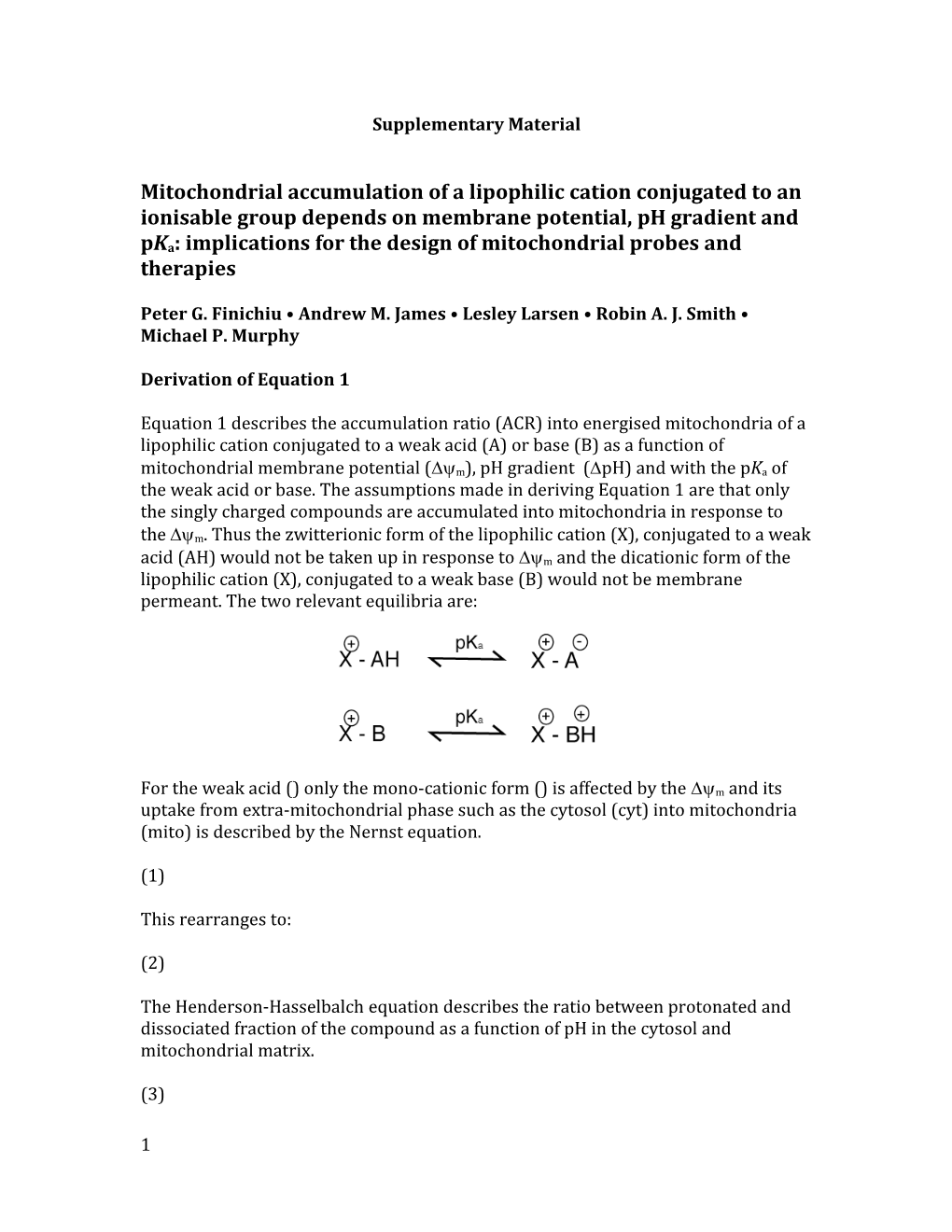# Peter G. Finichiu Andrew M. James Lesley Larsen Robin A. J. Smith Michael P. MurphySupplementary Material

Mitochondrial accumulation of a lipophilic cation conjugated to an ionisable group depends on membrane potential, pH gradient and pKa: implications for the design of mitochondrial probes and therapies

Peter G. Finichiu • Andrew M. James •Lesley Larsen•Robin A. J. Smith • Michael P. Murphy

Derivation of Equation 1

Equation 1 describes the accumulation ratio (ACR) into energised mitochondria of a lipophilic cation conjugated to a weak acid (A) or base (B) as a function of mitochondrial membrane potential (m), pH gradient (pH) and with thepKaof the weak acid or base. The assumptions made in deriving Equation 1 are that only the singly charged compounds are accumulated into mitochondria in response to them. Thus the zwitterionic form of the lipophilic cation (X), conjugated to a weak acid (AH) would not be taken up in response to mand the dicationic form of the lipophilic cation (X), conjugated to a weak base (B) would not be membrane permeant. The two relevant equilibria are:

For the weak acid () only the mono-cationic form () is affected by the m and its uptake from extra-mitochondrial phase such as the cytosol (cyt) into mitochondria (mito)is described by the Nernst equation.

(1)

This rearranges to:

(2)

The Henderson-Hasselbalchequationdescribes the ratio between protonated and dissociated fraction of the compound as a function of pH in the cytosol and mitochondrial matrix.

(3)

(4)

Substituting (2) into (4) gives:

(5)

The total concentration in each phase is:

(6) = +

(7) = +

Substitution of (3) into (6) yields

(8) = +

Substitution of (2) and (5) into (7) yields

(9) = +

The accumulation ratio (ACR) is defined by the total concentration of the compound inside the mitochondrion, i.e. , divided by the total concentration of the compound outside the mitochondrion, i.e. :

(10)

Therefore substitution of (8) and (9) into (10) yields

(11)

For the weak base () only the monocationic form () is affected by m and its uptake from the extramitochondrial phase such as the cytosol (cyt) into mitochondria (mito) is described by the Nernst equation.

(12)

This rearranges to:

(13)

The Henderson-Hasselbalchequationdescribes the ratio between protonated and dissociated fraction of the compound as a function of pH in the cytosol and mitochondrial matrix.

(14)

(15)

Substituting (13) into (15) gives:

(16)

The total concentration in each phase is:

(17) = +

(18) = +

Substitution of (14) into (17) yields

(19) = +

Substitution of (13) and (16) into (18) yields

(20) = +

The accumulation ratio (ACR) is defined by the total concentration of the compound inside the mitochondria () divided by the total concentration of the compound outside the mitochondrion ():

(21)

Therefore substitution of (19) and (20) into (21) yields

(22)

Summarising (11) and (22) the general accumulation ratio for a lipophilic cation conjugated to a weak acids or base is given byEquation 1where i is +1 for acid and -1 for base:

Equation 1

1